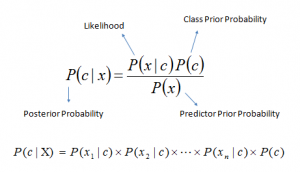/  Top Machine learning interview questions and answers   /  What are the Advantages and Disadvantages of Naïve Bayes Classifier?## What are the Advantages and Disadvantages of Naïve Bayes Classifier?

### Advantages of Naive Bayes

1. When assumption of independent predictors holds true, a Naive Bayes classifier performs better as compared to other models.

2. Naive Bayes requires a small amount of training data to estimate the test data. So, the training period is less.

3. Naive Bayes is also easy to implement.

### Disadvantages of Naive Bayes

1. Main imitation of Naive Bayes is the assumption of independent predictors. Naive Bayes implicitly assumes that all the attributes are mutually independent. In real life, it is almost impossible that we get a set of predictors which are completely independent.

2. If categorical variable has a category in test data set, which was not observed in training data set, then model will assign a 0 (zero) probability and will be unable to make a prediction. This is often known as Zero Frequency.